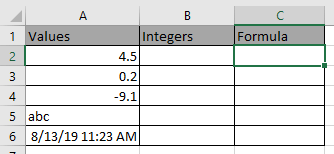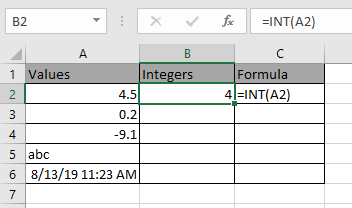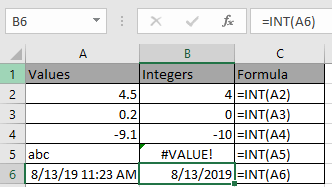# How to use the Excel INT function

The INT function returns the Integer part of the value.
Syntax:

=INT (number)

The function returns a value error if any text is input as argument.

Let’s understand this function using it an example.Use the formula:

=INT(A2)Copy the formula in other cells to get the integer part from the value.Some observational results

1. =INT(-9.1) returns -10, use TRUNC function to get the -9 as a result.
2. =INT(abc) returns the value error.
3. =INT(date & time) returns date as value. Excel considers the date as an integer and in date numbers and time part in decimals. To get time for new cell use (date & time_ value) - (date_value).

Hope you understood how to use Excel INT function in Excel. Explore more articles on Excel mathematical function here. Please feel free to state your query or feedback for the above article.

Related Articles:

How to use the Excel TRUNC Function

How to Use Round function in Excel

Popular Articles:

How to use the VLOOKUP Function in Excel

How to use the COUNTIF function in Excel 2016

How to Use SUMIF Function in Excel

Terms and Conditions of use

The applications/code on this site are distributed as is and without warranties or liability. In no event shall the owner of the copyrights, or the authors of the applications/code be liable for any loss of profit, any problems or any damage resulting from the use or evaluation of the applications/code.